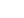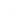ALWAYS POST BLESSEDWAP.XTGEM.COM ON YOUR FACEBOOK ,TWITTER AND 2GO STATUS! IF YOU LOVE BLESSEDWAP AND ENJOY UNLIMITED SERVICES HERE!
»Forum Smilies
:( =:DD =:D =:o =0_0 =:P =B) =>:( =O_O =>_> =-_- =:-( =:-) =^^ =;) =O:-) =:p =;-D =:*( =]:-> =(y) =<3 =:* =:-)) =[:) =[:-) =:-O =?-| =:aggressive: =:bomb: =:bye: =:celebrate: =:rolleyes: =:rose: =:secret: =:shy: =:sick: =:sorry: =:wack: =:wacko: =:yay: =3.231.217.107Advertise Your Site For Free! Design Your Site Here!Today's Visitors 1